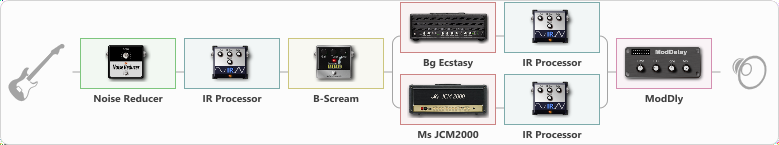# guitar solo-3

Discussion in 'ToneLib-GFX presets' started by carlinos3, Jun 13, 2022.

1. guitar solo-3

Effects chain:Effect: "Noise Reducer" (Dynamics / Filter), active - "yes"
{
"Sens" = 61
"Mode" = Hard
}

Effect: "IR Processor" (Cabinets), active - "yes"
{
"IR" = PRE-3_dc
"Low Cut (Hz)" = 0
"Hi Cut (kHz)" = 20.0
"Mix" = 100
"Level (dB)" = 14
}

Effect: "B-Scream" (Overdrive / Distortion), active - "yes"
{
"Drive" = 34
"Tone" = 75
"Level" = 100
}

Effect: "Splitter" (Dynamics / Filter)
{
"A-Bypass" = Off
"A-Pan" = 0
"A-Level" = 55
"B-Bypass" = Off
"B-Pan" = 0
"B-Level" = 57
"Width" = 0

'A' branch:
{

Effect: "Bg Ecstasy" (Amp simulators), active - "yes"
{
"Gain" = 100
"Bass" = 83
"Middle" = 50
"Treble" = 50
"Presence" = 73
"Master" = 100
"Level (dB)" = 7
}

Effect: "IR Processor" (Cabinets), active - "yes"
{
"IR" = imp-feb-2_dc
"Low Cut (Hz)" = 0
"Hi Cut (kHz)" = 20.0
"Mix" = 100
"Level (dB)" = 4
}
}
'B' branch:
{

Effect: "Ms JCM2000" (Amp simulators), active - "yes"
{
"Gain" = 100
"Bass" = 76
"Middle" = 50
"Treble" = 50
"Presence" = 50
"Master" = 100
"Level (dB)" = 5
}

Effect: "IR Processor" (Cabinets), active - "yes"
{
"IR" = charv.1_dc
"Low Cut (Hz)" = 0
"Hi Cut (kHz)" = 20.0
"Mix" = 100
"Level (dB)" = 0
}
}
}

Effect: "ModDly" (Delay), active - "yes"
{
"Time" = 508
"Feedback" = 23
"Tone" = 66
"Speed" = 1.7
"Mix" = 49
}

Note: You will need to download and install the ToneLib-GFX software to use the preset.

#### Attached Files:

• ###### guitar_solo-3.tlgfx
File size:
106.7 KB
Views:
1,827
aristokraft likes this.